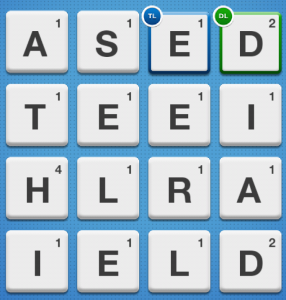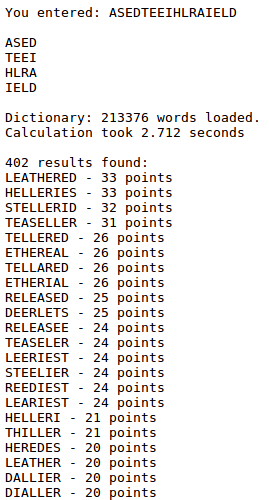# kleemans.ch

## Boggle

January 20, 2013

I digged out a script which I wrote some months ago, for finding the best words in Boggle / Scramble / Ruzzle etc. With ca. 210k words in a dictionary, each word is checked if it can be represented on the board. From all possible words, their values are calculated and then printed in descending order.

Sample grid:My script:Any multipliers (double/triple word/letter) are not taken into account. But at least you should manage to get the "the ultimate move" achievement in Ruzzle.

## Code

The code basically uses a simple search algorithm, exploring the possible neighbor letters and checking if they're in the dictionary.

All resulting entries are then scored based on letter value, and the entries are sorted top down, to give the most valuable ones first.

``````for word in words:
# speed & correctness
if len(word) < 2:
continue

possible = True
promising = True
starting_field = '0'
hist = starting_field

for letter in word:
if letter not in grid:
possible = False

while possible:
l = len(hist)
last_number = '0'
if l > 1:
last_number = int(hist[l-2:l-1], 16)
this_number = int(hist[l-1:], 16)

if grid[this_number] == word[l-1] and promising: # letter is correct & promising
if l == len(word): # if last letter ist correct, word is valid
log("> Letter is valid, word found!")
matches.append(word)
possible = False
else: # checking if adding new number is valid
this_index = len(graph[rd[this_number]])
for i in range(0, this_index):
new_number = str(graph[rd[this_number]][i])
log("new number: "+new_number)
if new_number not in hist: # if field not in use
hist += new_number
break
possible = False # dead end
else: # letter not correct
promising = True
log("> Letter not correct or not promising")
if len(hist) == 1: # change starting field
start = int(hist, 16)
if start == 15: # last possibility reached
log("> F reached with no possibilities")
possible = False
else:
hist = rd[int(hist, 16) + 1] # increment starting field
else: # not starting field
# get index
line = graph[rd[last_number]]
log("> calculating index in "+str(line))
index = "lulz"
for j in range(0, len(line)):
if line[j] == rd[this_number]:
index = j
log("> index found: " + str(j))

last_index = len(graph[rd[last_number]])
incremented = False
log("> looping from "+ str(index) + " to " + str(last_index))
for i in range(index, last_index):
log("> possibilities: " + str(graph[rd[last_number]]))
new_number = graph[rd[last_number]][i]
log("> checking " + new_number + " = " + grid[int(new_number, 16)] + " for adding...")
if new_number not in hist: # only if field not already in use
hist = hist[:len(hist)-1] + new_number
incremented = True
break
if not incremented: # else go back one step
log("> not incremented, go back one step")
if last[:len(word)-1] == hist: # check if last possibility
log("> breaking, last possibility: " + hist)
possible = False
else:
hist = hist[:l-1]
promising = False

# calculate most valuable matches
matches_count = len(matches)
l = dict(zip(matches, [val(n) for n in matches]))

# get a sorted instance of dict (list of tuples)
l = sorted(l.iteritems(), key = operator.itemgetter(1), reverse=True)

# Output
print "\n", matches_count, "results found: "

for i in l:
print i, "-", i, "points"
``````

You can find the whole source code (including an English dictionary, which is necessary) scramble-best-words.